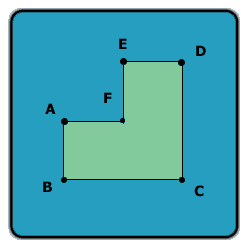All about flooble | fun stuff | Get a free chatterbox | Free JavaScript | Avatarsperplexus dot infoA different angle (Posted on 2003-10-10)Look at this shape:Assume AB = AF = FE = ED and BC = CD, and all the angles in the shape are 90 degrees.

Let A be the area of this shape (in cm^2), and P -- the perimeter of this shape (in cm).

If A - P is 60, what is the length of line AC?

 See The Solution Submitted by Lewis Rating: 1.9167 (12 votes)Comments: ( Back to comment list | You must be logged in to post comments.)simply put| Comment 3 of 23 |Let x be the length AB, AF, etc.
Then, the perimeter P = 8x, and the area of the figure A = 3x².
A - P = 60
3x² - 8x = 60
3x² - 8x - 60 = 0
(3x + 10)(x - 6) = 0
x = 6, -10.
Obviously, the distance in question must be positive, so x= 60.

Then, AC is the hypotenuse of a right triangle with legs 6 and 12.
Thus, AC² = 6² + 12²
AC = √180
AC = 6√5
AC ~= 13.4164
 Posted by DJ on 2003-10-10 09:20:37Please log in:

 Search: Search body:
Forums (5)